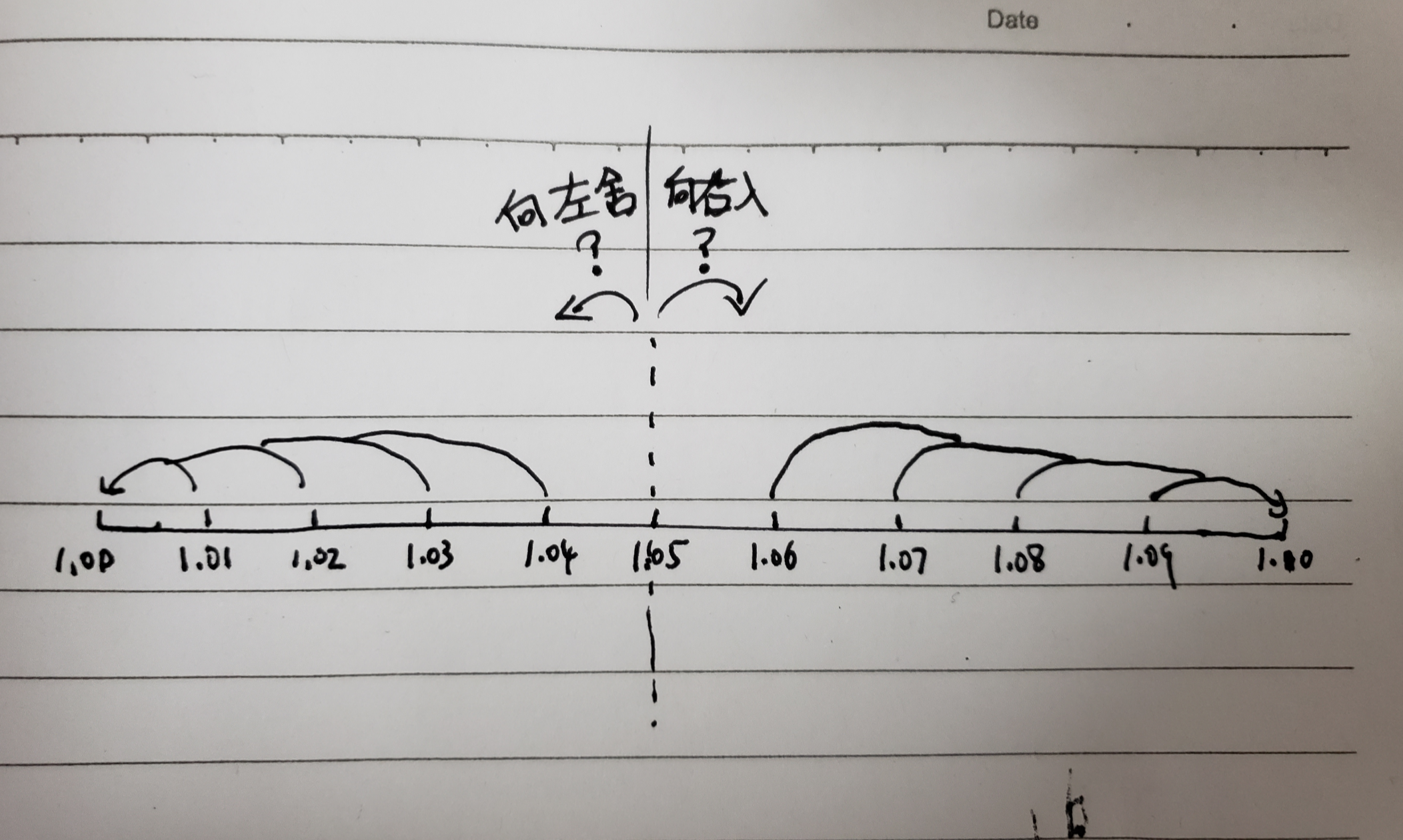2019/04/10 10:10

# 二、JS 与 精度

### 1、精度处理

• 1、小数才会涉及精度的概念
• 2、小数的（存储和）运算涉及 JS 的`精度处理`

### 2、精度丢失

``````0.1 + 0.2
0.30000000000000004

0.3 - 0.1
0.19999999999999998

0.1 * 0.1
0.010000000000000002

0.3 / 0.1
2.9999999999999996
``````

##### （1）问：精度丢失会引发什么问题？

• 1、让判断等于（`===`）的逻辑出错。比如让 `0.1 + 0.2 === 0.3``false`
• 2、让本来可以预想到的结果精度变的特别大，小数点后位数特别长。比如若要前端显示，会特别难看。
##### （2）问：为什么会出现精度丢失？

JS 采用 `IEEE 754` 标准的 64 位双精度浮点数表示法，这个标准是20世纪80年代以来最广泛使用的浮点数运算标准，为许多CPU与浮点运算器所采用，也被很多语言如 java、python 采用。

##### （3）问：怎么避免精度丢失？

<br>

• Math.js
• decimal.js
• big.js
• bignumber.js

<br>

``````console.log(parseFloat((0.3 + 0.1).toFixed(1))) // 0.4
``````

# 三、JS 与 近似计算方法

• 精度计算
• 精度丢失

### 1、四舍五入

##### （2）Math.round()

``````Math.Round(2.4) // 2
Math.Round(2.5) // 3
``````
##### （3）_.round() —— lodash

lodash 的这个方法，我看了源码，底层也是调用的 Math.round()，只是加了一些额外功能，比如第二个参数，可以指定四舍五入的精度。

``````const _ = require('lodash');
_.round(1.04, 1) //1
_.round(1.05, 1) //1.1
``````
##### （4）四舍五入真的公平吗？【重点】

``````const _ = require('lodash');
console.log(_.round(1.01, 1)) //1 （我亏了0.01）
console.log(_.round(1.02, 1)) //1 （我亏了0.02）
console.log(_.round(1.03, 1)) //1 （我亏了0.03）
console.log(_.round(1.04, 1)) //1 （我亏了0.04）
console.log(_.round(1.05, 1)) //1.1 （我赚了了0.05）
console.log(_.round(1.06, 1)) //1.1 （我赚了0.04）
console.log(_.round(1.07, 1)) //1.1 （我赚了0.03）
console.log(_.round(1.08, 1)) //1.1 （我赚了0.02）
console.log(_.round(1.09, 1)) //1.1 （我赚了0.01）
``````### 3、toFixed

toFixed() 部分符合银行家舍入的规则。

##### （3）五后为空看奇偶，五前为偶应舍去，五前为奇要进一

``````//                           //toFixed结果  //银行家舍入结果
console.log(1.05.toFixed(1)) //1.1（+0.05） 1.0（-0.05）
console.log(1.15.toFixed(1)) //1.1（-0.05） 1.2（+0.05）
console.log(1.25.toFixed(1)) //1.3（+0.05） 1.2（-0.05）
console.log(1.35.toFixed(1)) //1.4（+0.05） 1.4（+0.05）
console.log(1.45.toFixed(1)) //1.4（-0.05） 1.4（-0.05）
console.log(1.55.toFixed(1)) //1.6（+0.05） 1.6（+0.05）
console.log(1.65.toFixed(1)) //1.6（-0.05） 1.6（-0.05）
console.log(1.75.toFixed(1)) //1.8（+0.05） 1.8（+0.05）
console.log(1.85.toFixed(1)) //1.9（+0.05） 1.8（-0.05）
console.log(1.95.toFixed(1)) //1.9（-0.05） 2.0（+0.05）
//                           //总计(+0.1)   //总计(0)
``````

### 4、其他 近似计算 函数

• Math.ceil()：向上舍入（取整）
• Math.floor()：向下舍入（取整）
• 等等……

0
0 收藏

### 作者的其它热门文章0 评论
0 收藏
0